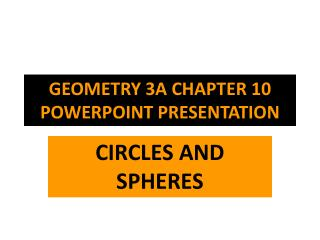DownloadDownload PresentationGEOMETRY 3A CHAPTER 10 POWERPOINT PRESENTATION

# GEOMETRY 3A CHAPTER 10 POWERPOINT PRESENTATION

Télécharger la présentation## GEOMETRY 3A CHAPTER 10 POWERPOINT PRESENTATION

- - - - - - - - - - - - - - - - - - - - - - - - - - - E N D - - - - - - - - - - - - - - - - - - - - - - - - - - -
##### Presentation Transcript

1. GEOMETRY 3ACHAPTER 10 POWERPOINT PRESENTATION CIRCLES AND SPHERES

2. LEARNING TARGETS • AFTER YOU COMPLETE THIS CHAPTER, YOU WILL BE ABLE TO: • IDENTIFY FORMULAS FOR: CIRCUMFERENCE, DIAMETER, RADIUS • SOLVE PROBABILITY PROBLEMS • DETERMINE THE AREA OF A CIRCLE • DEFINE TRIGONOMETRIC RATIOS AND USE THEM • DETERMINE VOLUME AND SURFACE AREA OF A SPHERE

3. CIRCLE - VOCABULARY • CIRCLE: SET OF POINTS AT THE SAME DISTANCE FROM A GIVEN POINT • RADIUS: (r) DISTANCE BETWEEN THE CENTER OF A CIRCLE AND ANY POINT ON THE CIRCLE • CHORD: LINE SEGMENT JOINING TWO POINTS ON A CIRCLE • DIAMETER: (d) A CHORD THAT PASSES THROUGH THE CENTER OF A CIRCLE • CIRCUMFERENCE: (c)THE COMPLETE LENGTH AROUND A CIRCLE • QUADRANT: ONE-FOURTH OF A CIRCLE

4. WHAT THAT LOOKS LIKE

5. CHORDS

6. THE RATIO PI • Pi = 22/7 or 3.14 • Its symbol is:

7. Circle Formulas • Area:

8. Circumference • Circumference of a circle:

9. 2 formulas (if you know the radius or the diameter)

10. Estimation of Area of Circle • Area estimation formula: area of a circle = 3r² • Where r = radius • Approximating the area of a circle:

11. Symbol for approximately:

12. Area and Probability • Probability means the chances or likelihood of an event happening. • Suppose you pick any point inside the circle, what is the probability of picking a point in the top semi-circle? 1 out of 2 • If a circle is split into four quadrants, what is the probability of landing on a quadrant with an even number: 1 out of 2

13. Area of a Circle • Area = πr² (use when you know the radius) • Area = ¼πd² (use when you know the diameter)

14. ADDITIONAL CIRCLE VOCABULARY SECTOR: THE AREA ENCLOSED WITHIN A CENTRAL ANGLE OF A CIRCLE CENTRAL ANGLE: AN ANGLE WITH ITS VERTEX AT THE CENTER OF A CIRCLE AND THE CIRCLE’S RADII (PLURAL OF RADIUS) AS ITS SIDE ARC: A PORTION OF A CIRLCE BOUNDED BY TWO DISTINCT POINTS ON THE CIRCLE

15. WHAT DOES THAT LOOK LIKE?

17. Central Angles

18. ARCS

19. More Circle Vocabulary • Inscribed Angle: An angle formed by two chords that intersect on the circle. • Intercepted Arc: The arc of a circle within an inscribed angle. • Tangent: A line that touches but does not intersect a circle. • Point of Tangency: The point where the tangent touches the circle

20. Tangent & Point of Tangency

21. Inscribed Angle & Intercepted Arc

22. CIRLCE VOCABULARY, CONTINUES… • Perpendicular Bisector: A set of points equidistant from two given points. • Equidistant: At an equal distance. • Locus of Points: A set of points that satisfy a certain condition.

23. Perpendicular Bisector

24. Equidistant Points

25. Locus of Points

26. And the Circle Vocabulary Just Keeps Coming!!!! • Circumcircle: A circle that passes through three vertices of a triangle. • Circumcenter: Center of a circumcircle and located at the intersection of the perpendicular bisectors of any two sides of a triangle. • Angle Bisector: Locus of points equidistant from the sides of an angle. • Incircle: A circle inside a triangle and tangent to each of the triangle’s sides.

27. What That Looks Like! • Circumcircle & Circumcenter:

28. Angle Bisectors

29. Incircle

30. Sine, Cosine, Tangent • Unit Circle: Circle whose radius is one. • Sine (sin): for an angle of a right triangle, not the right angle, the ratio of the length of the opposite leg divided by the length of the hypotenuse. • Cosine (cos): for an angle of a right triangle, not the right angle, the ratio of the length of the adjacent leg divided by the length of the hypotenuse. • Tangent (tan): for an angle of a right triangle, not the right angle, the ratio of the length of the opposite side divided by the length of the adjacent leg.

31. Trigonometry • Trigonometry – The branch of mathematics dealing with the relation between the sides and angles of triangles. For right triangles.

32. The Sphere • Sphere: Locus of points in space equidistant from a fixed point. • Great Circle: Circle on a sphere whose center is the center of the sphere and whose radius equals the radius of the sphere. • Hemisphere: Half of a sphere. • Poles: Endpoints of the diameter of a sphere. • Formulas: SA = 4πr² Volume: 4/3πr³

33. What the Parts of a Sphere Look Like • Great Circle:

34. Hemispheres

35. Poles